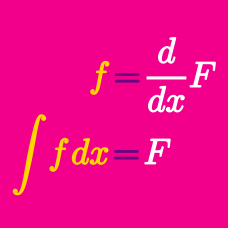Calculus

# Antiderivative and Indefinite Integration

If two polynomial functions $f(x)$ and $g(x)$ satisfy $\frac{d}{dx}\{f(x)+g(x)\}=7, \frac{d}{dx}\{f(x)g(x)\}=12x-1, f(0)=0, g(0)=-1,$ what is $f(1)-g(1)?$

If $f(x)=\int \left\{\frac{d}{dx} \left(x^3-5x^2+18x\right)\right\} dx \text{ and } f(1)=2,$ what is $f(3)?$

If $a ,b,$ and $c$ are constants satisfying $\int \left( 12x^3 +ax-9\right)dx=bx^4+3x^2+cx+C,$ what is the value of $a+b+c?$

Details and assumptions

$C$ is the constant of integration.

If $a, b$ and $c$ are constants and $\int (18x^2+ax+1)dx=bx^3+8x^2+cx+C,$ where $C$ is the constant of integration, what is $a+b+c?$

If $f(x)$ satisfies $\int (2x+1)f(x)dx = x^4-2x^3-2x^2+C,$ what is the value of $f(5)?$

Details and assumptions

$C$ is the constant of integration.

×